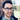# Two Number Sum

algorithms
personal
go
python

May 27, 2019

In this article we discuss a very common, and fairly simple algorithm problem, the Two Number Sum.

The challenge is as follows:

Write a function that takes in a non-empty array of distinct integers and an integer representing a target sum. If any two numbers in the input array sum up to the target sum, the function should return them in an array, in sorted order. If no two numbers sum up to the target sum, the function should return an empty array. Assume that there will be at most one pair of numbers summing up to the target sum.

Example

Sample input: [3, 5, -4, 8, 11, 1, -1, 6], 10

Sample output: [-1, 11]

## Non-optimal solution

``````def twoNumberSum(array, targetSum):
if (len(array) <= 0):
return []
for i in range(len(array)):
for j in range(i+1, len(array)):
if array[i] + array[j] == targetSum:
return sorted([array[i], array[j]])
return []``````

We can solve this problem using a non-optimal and more “brute” force approach, where we compare every pair of integers in the input, comparing with the target value. This would imply the following constraints:

Time: O(n^2) - we have to iterate over the list of size n twice

Memory - O(1) - we compare the values on each iteration, never storing results until the end.

This could be sufficient if we are dealing with a reasonable small value of n, and have memory space constraints. However, as we need to scale this algorithm to larger n, and potentially enable the distribution across a number of machines, we need to think about more effective solutions.

## Optimization through memoization

Memoization employs a basic technique of storing results of previous function calls, preventing unnecessary duplicated calculations. We will use this approach to store the results of previous two number sum comparisons to then lookup from when evaluation future comparisons.

``````def twoNumberSum(array, targetSum):
if (len(array) <= 0):
return []
previousNum = []
for i in range(len(array)):
y = targetSum-array[i]
if y in previousNum:
return sorted([array[i],y])
previousNum.append(array[i])
return []``````

So now we’re populating the `previousNum` table, which gives us the “memory” or ability to evaluate whether any of the previously encountered numbers will be suitable to reach `targetSum`. Effectively reducing the second iteration we were doing in the first approach.

Time: O(n) - we still have to iterate over the list once, and insertion and lookups are O(1) for a hash table

Memory: O(n) - we are building a table of potentially n items, which we then lookup

So the trade-off in this solution is to make use of memory to minimise additional computations, utilising the properties of a hash table which gives us `O(1)` insert and lookup capabilities. The key considerations that go into the trade-off would be what are you limited by in terms of computational resources, is it memory space or compute space, which one is more expensive?. An interesting philosophical extreme to consider is the correlation of compute space with time, and memory space with mass. Do you have infinite time, or infinite mass? An interest physical conundrum. If we look to some of the strange objects in our universe, such as a blackhole, which has infinite density at its singularity, as it collapses a given mass into zero volume, effectively dividing by zero - where `Density = Mass/Volume`. What does this imply about the memory space of a blackhole?

Fundamentally information cannot be destroyed, it can only be converted or transfer to the surrounding space, as described in the No-Hiding Theorem and Black hole information paradox. Some food for thought.

## structured input - further optimisations

If we assume that this list of numbers is in a sorted structure, then how can we take advantage of this property to further optimise the algorithm.

The usual answer is [Binary Search][https://en.wikipedia.org/wiki/Binary_search_algorithm], where we can now “search” the input efficiently when evaluating matches for the targetSum. In our scenario we won’t be doing binary search, but we will definitely be able to more effectively search or iterate through the numbers in the list.

``````def twoNumberSum(array, targetSum):
if (len(array) <= 0):
return []
L = 0
R = len(array) - 1
previousNum = []
while L < R:
total = array[L]+array[R]
if total == targetSum:
return [array[L],array[R]]
elif total > targetSum:
R -= 1
else:
L += 1
return []``````

With a known sorted list we can take advantage of the ordering to determine whether we should progress searching from the right side, or the left side, depending on whether the `total` is greater than or less than the `targetSum`.

If `total` is greater than `targetSum` then we can decrement the right index

If `total` is less than `targetSum` then we can increment the left index

This gives us the following cyclomatic complexity

Time: O(nlog(n)) - utilising the sorted structure, we can search faster than O(n^2) Space: O(1) - we don’t store any information during search

So the clear benefit here is we don’t need to consume memory and we still achieve a reasonable order of time complexity. Similar to the second solution, it comes down to what your resource costs are and goals.

Something to consider in this solution is how it would dramatically simplify the scaled implementation, where now we wouldnt need to manage anything relating to a lookup table, as used in `solution 2`. It identifies the importance of enabling the storage of data in a structured way that then facilitates solutions like that shown in `solution 3`. Where the costs associated with the structuring of ingested numbers can be decoupled from the task of searching them.Written by Ben Ebsworth, thoughts are their own and any material is representative of an attempt to self-educate and explore ideas and technology You should follow him on Twitter